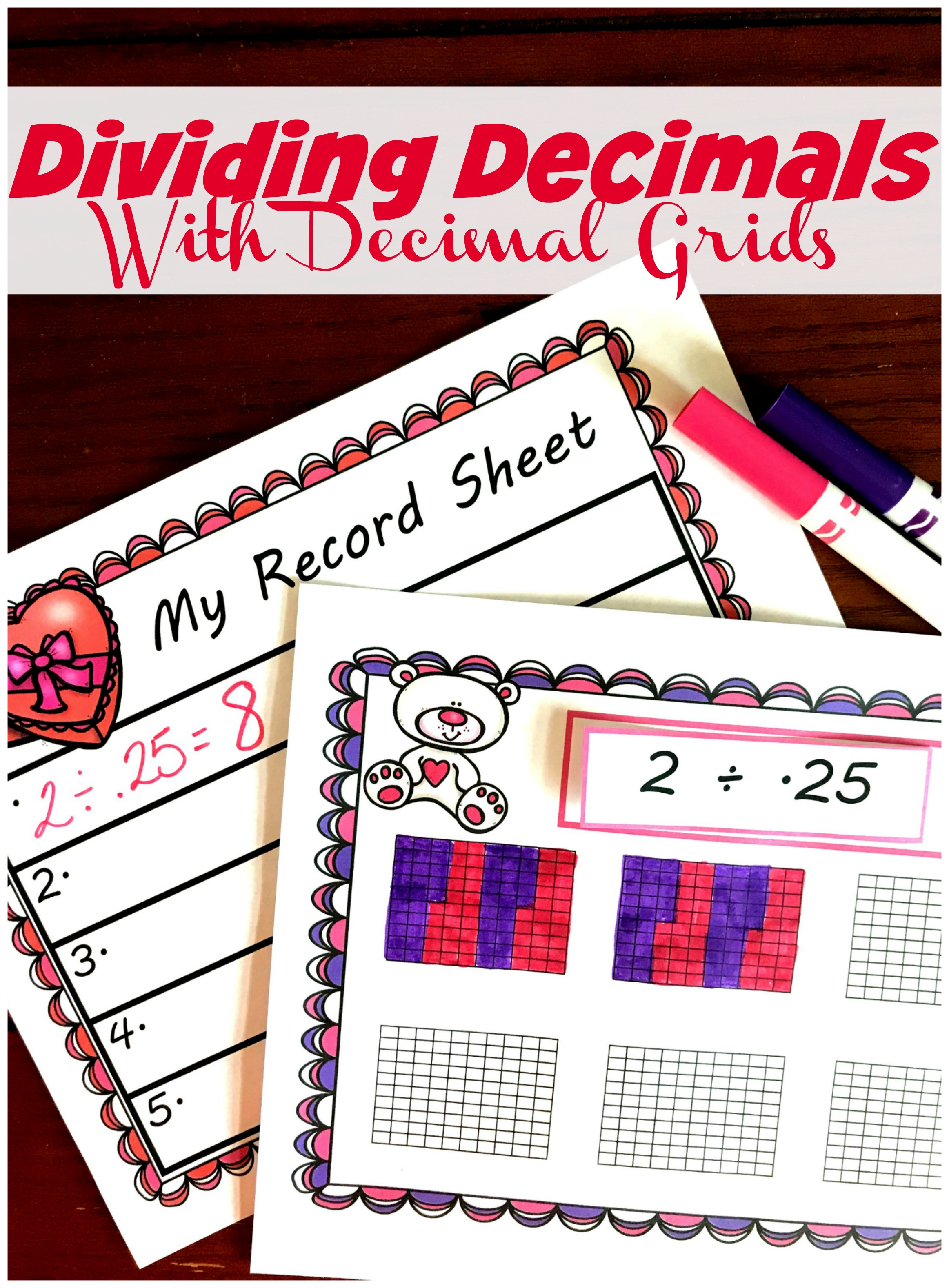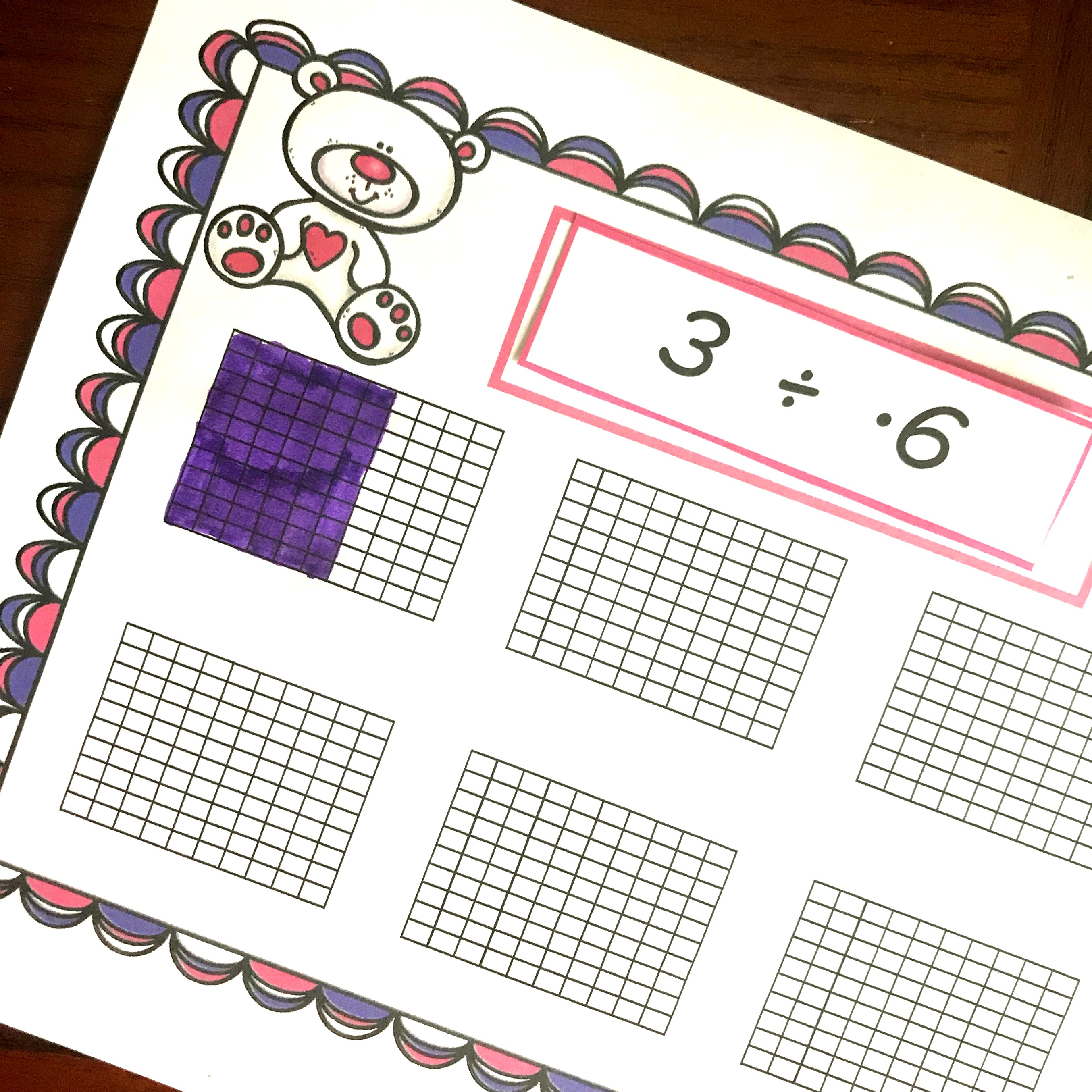Home » Math » FREE Valentine’s Day Dividing Whole Numbers by Decimals Worksheet

FREE Valentine’s Day Dividing Whole Numbers by Decimals Worksheet

You’ll love this free dividing whole numbers by decimals worksheet with a Valentine’s Day theme. Children solve division problems by coloring in decimal grids and then record the answer.Did you know that when you divide by decimals your answer will be larger than what you started with?

Yep, and this is quite confusing to our fifth and sixth graders. For most of their life when they divided up a number their answer is smaller, and now you are rocking their world with this new tidbit of info.

As always, we want our children to know WHY this happens. And this dividing whole numbers by decimals worksheet will help them see why!

Prep-Work

1. First, print off all the pages. Each child will need a record sheet and the coloring mat, but the children can share the expressions.
2. Next, laminate the coloring mat or place in a sheet protector.
3. Now, cut out the expressions.
4. Finally, gather up dry erase markers and pencils and you are ready to go.

Dividing Whole Numbers by Decimals Worksheet

So how do we use these pages?

The children begin by drawing an expression. In this example, they drew 3 ÷ .6!

This tells us that we are going to use three decimal grids and divide them up into six-tenths.As you can see here, this student began by coloring in six-tenths, and they will continue to do this until they have colored in three whole decimal grids.

Once that is done it is time to find the answer. How many equal groups do we have…..and the answer is five.

3 ÷ .6 = 5Why Modeling is Important

Children are used to their quotient being smaller than what they started with. If I’m dividing 10 into 2 equal groups my answer will be smaller than 10. We all know that there were only be 5 in each group.

But when we divide with fractions and decimals our quotient is larger than our dividend…..and our big kiddos need to see why this is.

When we have them model it out, they can see why the quotient or answer is larger than the dividend.

Modeling is a great place to begin your division unit, and I hope your children enjoy this activity.

You’ve Got This,

Rachel

You may also like:

Multiplying decimals with modelsHow to Model Decimals